Homework Help Question & Answers

# Two masses are hung from an ideal pulley. The 1.2 kg mass is on the ground...

Two masses are hung from an ideal pulley. The 1.2 kg mass is on the ground while the 1.5 kg mass is 4 m above the ground. Find the speed of the masses when they pass each other.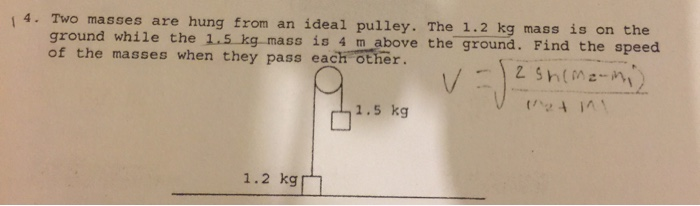#### Homework Answers

Answer #1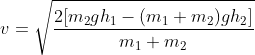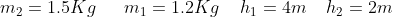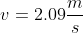Know the answer?
Your Answer:

#### Post as a guest

Your Name:

What's your source?

#### Earn Coin

Coins can be redeemed for fabulous gifts.

Not the answer you're looking for? Ask your own homework help question. Our experts will answer your question WITHIN MINUTES for Free.
Similar Homework Help Questions
• ### 4. Blocks of masses 11.0 kg and 25.0 kg hang on opposite sides of a pulley...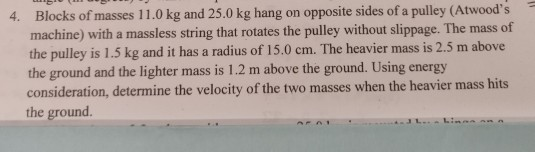4. Blocks of masses 11.0 kg and 25.0 kg hang on opposite sides of a pulley (Atwood's machine) with a massless string that rotates the pulley without slippage. The mass of the pulley is 1.5 kg and it has a radius of 15.0 cm. The heavier mass is 2.5 m above the ground and the lighter mass is 1.2 m above the ground. Using energy consideration, determine the velocity of the two masses when the heavier mass hits the ground....

• ### 1. Two masses are connected with a rope via a pulley. Mass m1 is 4.00 kg...

1. Two masses are connected with a rope via a pulley. Mass m1 is 4.00 kg and initially at rest on the floor. Mass m2 is 7.00 kg and initially is held at rest 2.00 m above the ground. Find the speed of m2 when it impacts the floor. 2. In each cycle of its operation, a heat engine expels 2,900 J of energy and performs 1,700 J of mechanical work. Find the thermal efficiency of the engine

• ### Two masses are suspended from a pulley. The pulley has a mass of 0.30 kg and...

Two masses are suspended from a pulley. The pulley has a mass of 0.30 kg and a radius of 0.22 m. Mass one is 0.50 kg and mass two is 0.70 kg. What is the acceleration of the system?

• ### Two boxes are connected to a rope, and the rope is hung over a pulley connected...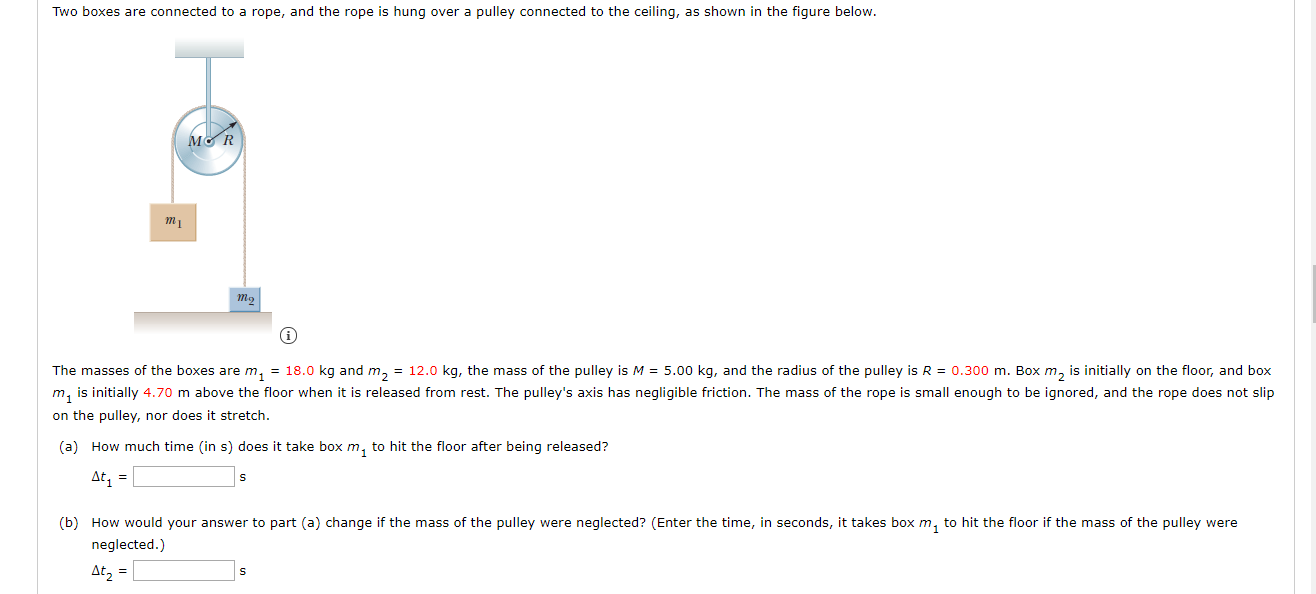Two boxes are connected to a rope, and the rope is hung over a pulley connected to the ceiling, as shown in the figure below. MCR mi The masses of the boxes are m, = 18.0 kg and m, = 12.0 kg, the mass of the pulley is M = 5.00 kg, and the radius of the pulley is R = 0.300 m. Box m, is initially on the floor, and box m, is initially 4.70 m above the floor...

• ### Two masses, mA = 34.0 kg and mB = 40.0 kg , are connected by a...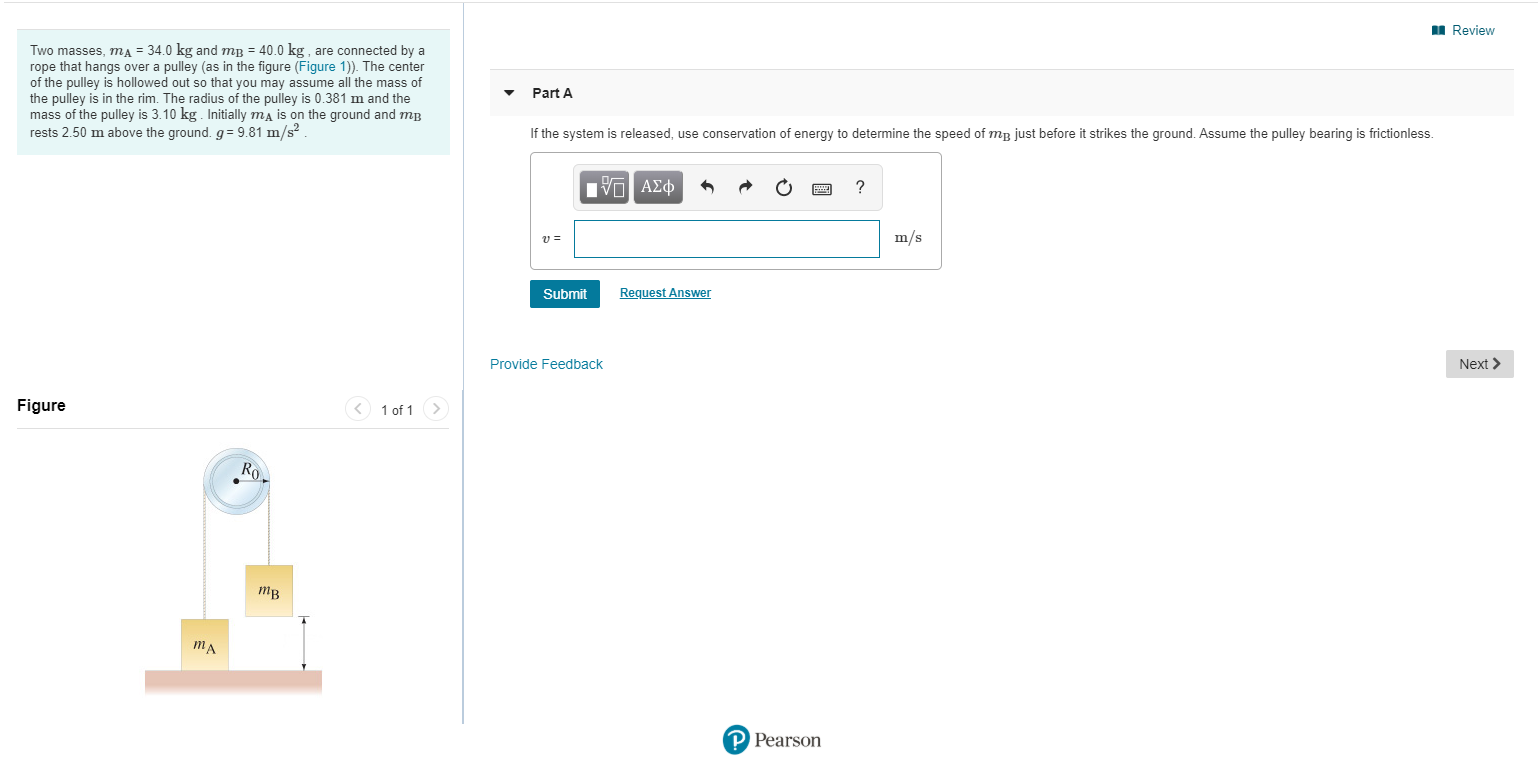Two masses, mA = 34.0 kg and mB = 40.0 kg , are connected by a rope that hangs over a pulley (as in the figure (Figure 1)). The center of the pulley is hollowed out so that you may assume all the mass of the pulley is in the rim. The radius of the pulley is 0.381 m and the mass of the pulley is 3.10 kg . Initially mA is on the ground and mB rests 2.50 m...

• ### Two blocks are connected to a string, and the string is hung over a pulley connected...

Two blocks are connected to a string, and the string is hung over a pulley connected to the ceiling, as shown in the figure below. Two blocks, labeled m1 and m2, are connected to a string which is hung over a pulley connected to the ceiling. The pulley is of mass M and radius R. A block labeled m1 hangs suspended off the surface on the left side of the pulley. A block m2 is on the right side of...

• ### In the figure, two masses, each 24 kg, are at rest and connected by the pulley. t...continues

In the figure, two masses, each 24 kg, are at rest and connected by the pulley. the pulley is massless and frictionless. Use Energy and work to find. a) the speed ofthe masses after thay have moved 1.6 m assumming the surface is frictionless. b)the speed of the masses after they moved 1.6 m assumming the coefficient of kineticfriction between the inclined surface and the mass is 0.31. Angle is 30 degree.

• ### A mass m = 0.4 kg is hung from a pulley with mass M = 0.20...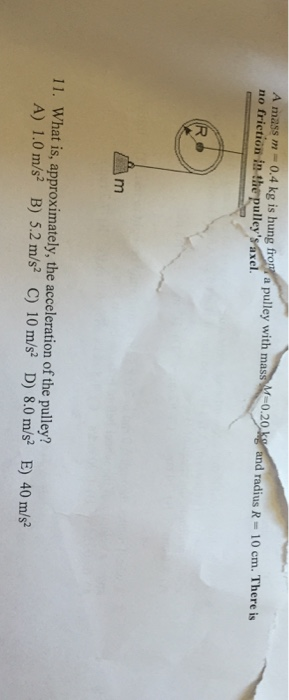A mass m = 0.4 kg is hung from a pulley with mass M = 0.20 kg and radius R = 10 cm. There is no friction in the pulley's axel. What is, approximately, the acceleration of the pulley? 1.0 m/s^2 5.2 m/s^2 10 m/s^2 8.0 m/s^2 40 m/s^2

• ### Two blocks of masses m_1 = 4 kg and m_2 = 5kg are connected by a...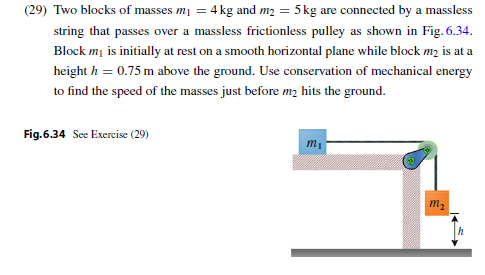Two blocks of masses m_1 = 4 kg and m_2 = 5kg are connected by a massless string that passes over a massless frictionless pulley as shown in Fig. 6.34. Block m_1 is initially at rest on a smooth horizontal plane while block m_2 is at a height h = 0.75 m above the ground. Use conservation of mechanical energy to find the speed of the masses just before m_2 hits the ground.